## Explain Conductivity of Metals:

Conductivity of Metals – Consider a conductor of length l metres and cross-sectional area A square metres, as shown in Fig. 6.27. Let the number of free electrons available per m3 of the conductor material i.e., electron density be n and E be the applied electric field. Due to the applied electric field, let axial drift velocity of the electrons be v metres/second.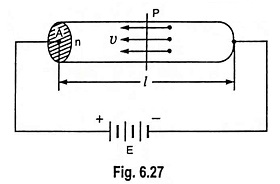The force F on the particle of charge q when an electric field E is applied is given as F = qE, so the force acting on an electron having charge e on it is given as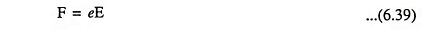Also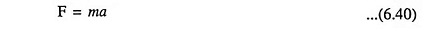where a is acceleration of electrons due to applied field E

Combining Eqs. (6.39) and (6.40), we have

Acceleration,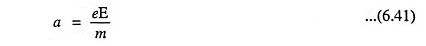Because of collisions of electrons during motion, the electrons will not get accelerated indefinitely. In fact, their velocity will fall to zero. If τ is the relaxation or collision time, the average velocity of electrons, known as drift velocity is given as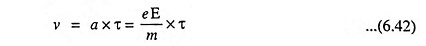Let I be the current flowing through the conductor on application of electric field E corresponding to drift velocity v. In time dt, the electrons will travel a distance vdt and the number of electrons crossing the cross-sectional area A in time dt will be contained in volume Avdt. Thus, total charge flowing through the section in time dt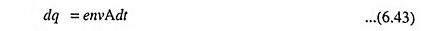Since the current in a conductor is defined as the rate of change of charge per unit time, current I is given as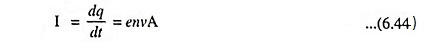The current density J, defined as the current per unit area is given as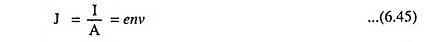The above equation may also be written as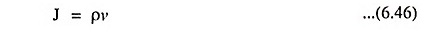where ρ is the charge density in coulombs/m3 = en

Substituting the value of v from Eq. (6.42) in above Eq. (6.45), we haveFor a particular material, the quantity ne2τ/m in above equation is constant at particular temperature and is known as electrical conductivity ‘σ’ of the material and thus

Current density,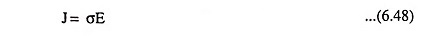So, the current density J is proportional to the Conductivity of Metals σ and the magnitude of applied electric field E. In terms of resistivity ρ, current density J is given as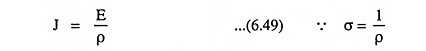We know that the resistance R of a conductor is given as R = ρ l/A where ρ is the resistivity of material and l is the length of conductor. Thus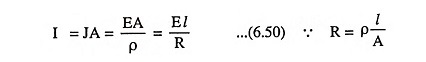Since E = V/l, the Eq. (6.50) may be written as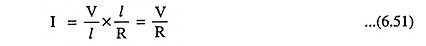which is nothing but ‘Ohms’ law.

Electrical Conductivity of Metals is also given as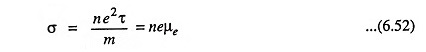where μe = eτ/m is the mobility acquired by electrons due to presence of electric field.

Using Eq. (6.42), the mobility of electrons can also be expressed as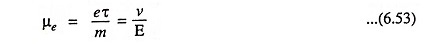Thus, the mobility of electron in the metal is defined as the steady-state drift velocity per unit electric field.

Let λ be the mean free path and v’ be the root mean square velocity of electrons, then the relaxation time (commonly known as mean time between collisions) τ is given as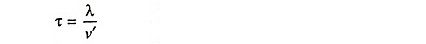But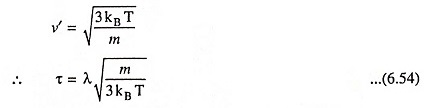Now the electrical conductivity σ can be expressed as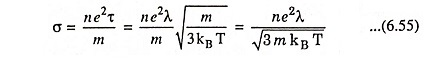Since electrical resistivity ρ is reciprocal of electrical Conductivity of Metals σ,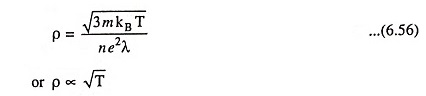Above result shows that the resistivity varies as √T whereas actually it is found to vary linearly with temperature.

Scroll to Top